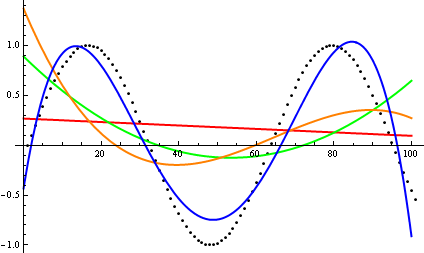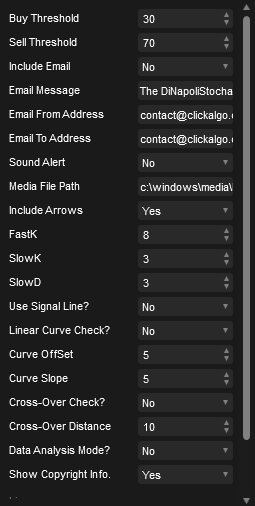Search
GBP
ENG

# cTrader DiNapoli Stochastic - Linear Curve Fitting

This version of the cTrader DiNapoli Stochastic has a smoother indicator and extra logic to match the curve of the lines to identify possible price reversal using linear curve fitting with Polynomial Interpolation. We also included many useful features like email, sound alerts, high and low thresholds.

£9.99
i h

This version of the cTrader DiNapoli Stochastic has a smoother indicator and extra logic to match the curve of the lines to identify possible price reversal with linear curve fitting. We also included many useful features like email, sound alerts, high and low thresholds.

This oscillator smoothing method leads to filtering out "noise" in the price movement. It is used in the strategies that are oriented to a standard stochastic. The stronger smoothing can lead to loss of an array of signals, so it is recommended to apply any trend indicator for more efficient use of the indicator.## Signal Line Cross-Over Check

This involves a very simple, but useful check on the distance between the signal and result lines using the cross-over distance setting when there is indecision in the price the two lines are very close together when there is a strong change direction the distance is higher.

## Linear Regression Curve Check

This is very useful when you want to match a specific type of curve which mostly show price reversals, it uses the Math.Net Numerics library described below for curve fitting. You can curve fit either the signal or the result line. The settings are Curve Offset and Curve Slope.

For analysis, you can use a setting called Data Analysis Mode which will show you historical curve values in the log file to help you match the values which hold the strongest weight. The video below will explain the features in more detail.## Math.NET Numerics

This assembly aims to expose algorithms and methods for numerical computations in software engineering, the areas it covers are special functions, linear algebra, probability models, random numbers, interpolation, integration, regression, optimization problems and much more.

## Curve Fitting: Linear Regression & Polynomial Interpolation

Regression is all about fitting a low order parametric model or curve data, so we can use it to make predictions on points not covered by the data. Both the data and the model are known, but we'd like to find the parameters that make the model good enough to the data according to some metric.

Curve fitting can involve either interpolation, where an exact fit to the data is required, or smoothing, in which a "smooth" function is constructed.## Data Analysis Mode

We have added a feature which will allow you to visually see the Linear Curve fitted values for the stochastic cross-over points so that you can compare this to the candle price action to help you increase the probability of a successful entry point.## Watch a Video Demonstration

The video has been uploaded to 1080p High Quality, so do not forget to set your U-Tube video quality to 1080p HD.

## Helpdesk Support

If you cannot find the help you need on the product support page then submit a support ticket.

Submit a Support Ticket

* Manual trading only - source code not available.

This version of the cTrader DiNapoli Stochastic has a smoother indicator and extra logic to match the curve of the lines to identify possible price reversal with linear curve fitting. We also included many useful features like email, sound alerts, high and low thresholds.

This oscillator smoothing method leads to filtering out "noise" in the price movement. It is used in the strategies that are oriented to a standard stochastic. The stronger smoothing can lead to loss of an array of signals, so it is recommended to apply any trend indicator for more efficient use of the indicator.## Signal Line Cross-Over Check

This involves a very simple, but useful check on the distance between the signal and result lines using the cross-over distance setting when there is indecision in the price the two lines are very close together when there is a strong change direction the distance is higher.

## Linear Regression Curve Check

This is very useful when you want to match a specific type of curve which mostly show price reversals, it uses the Math.Net Numerics library described below for curve fitting. You can curve fit either the signal or the result line. The settings are Curve Offset and Curve Slope.

For analysis, you can use a setting called Data Analysis Mode which will show you historical curve values in the log file to help you match the values which hold the strongest weight. The video below will explain the features in more detail.## Math.NET Numerics

This assembly aims to expose algorithms and methods for numerical computations in software engineering, the areas it covers are special functions, linear algebra, probability models, random numbers, interpolation, integration, regression, optimization problems and much more.

## Curve Fitting: Linear Regression & Polynomial Interpolation

Regression is all about fitting a low order parametric model or curve data, so we can use it to make predictions on points not covered by the data. Both the data and the model are known, but we'd like to find the parameters that make the model good enough to the data according to some metric.

Curve fitting can involve either interpolation, where an exact fit to the data is required, or smoothing, in which a "smooth" function is constructed.## Data Analysis Mode

We have added a feature which will allow you to visually see the Linear Curve fitted values for the stochastic cross-over points so that you can compare this to the candle price action to help you increase the probability of a successful entry point.## Watch a Video Demonstration

The video has been uploaded to 1080p High Quality, so do not forget to set your U-Tube video quality to 1080p HD.

## Helpdesk Support

If you cannot find the help you need on the product support page then submit a support ticket.

Submit a Support Ticket

* Manual trading only - source code not available.

Products specifications
 Find more at Manual
Product tags